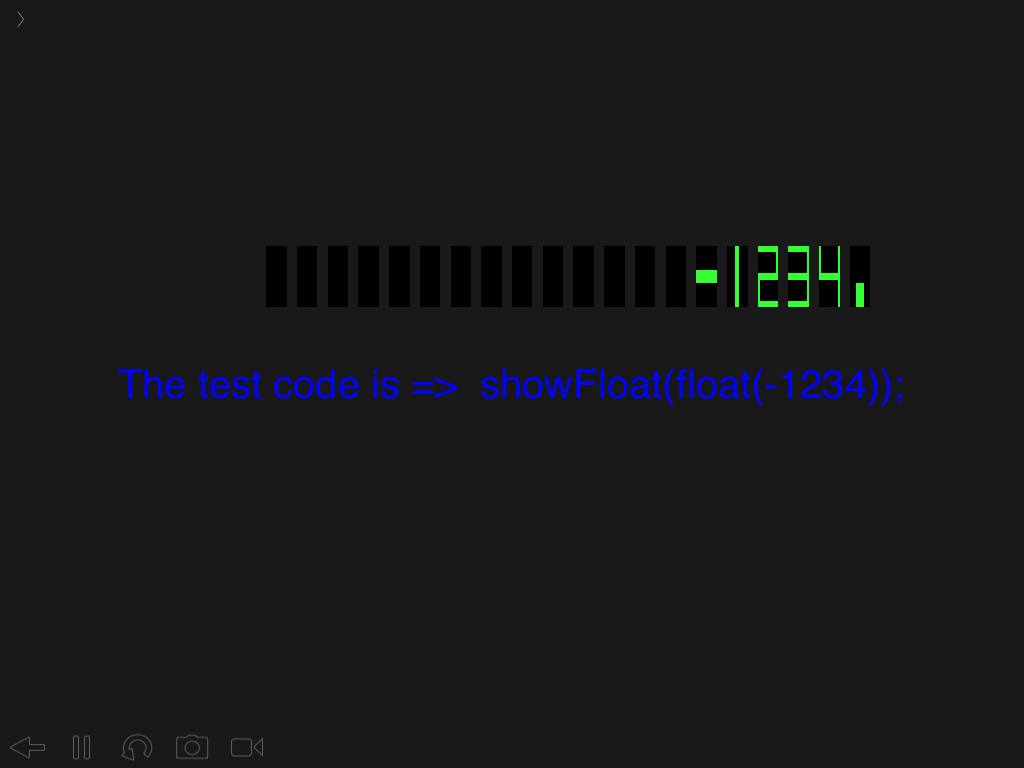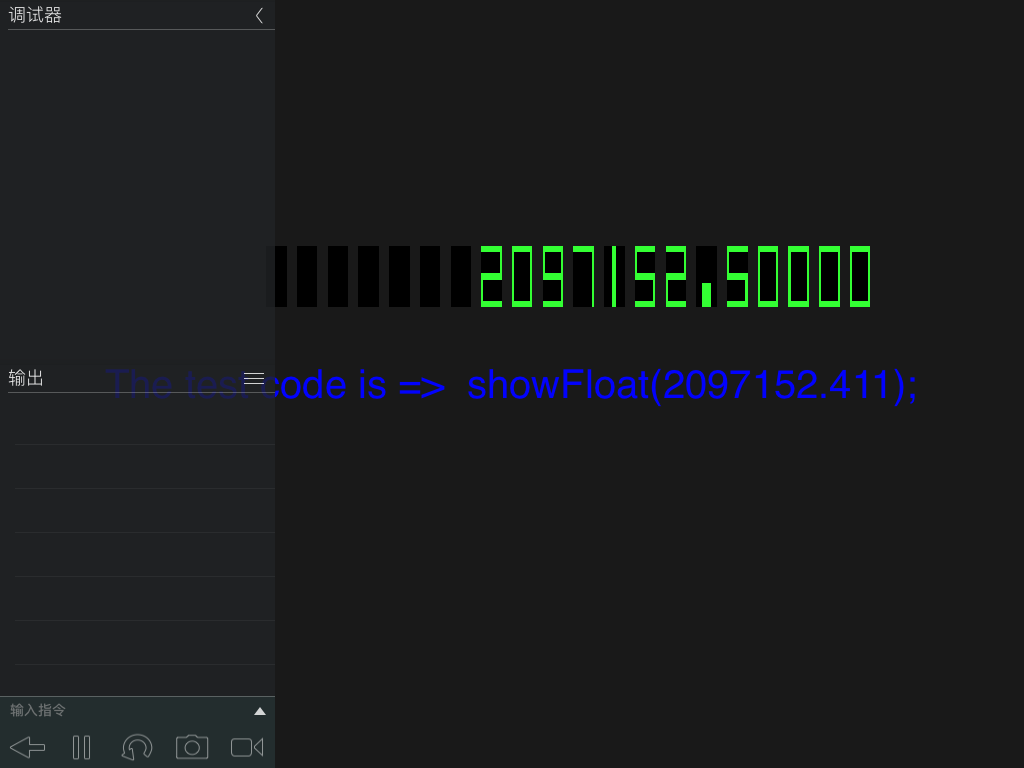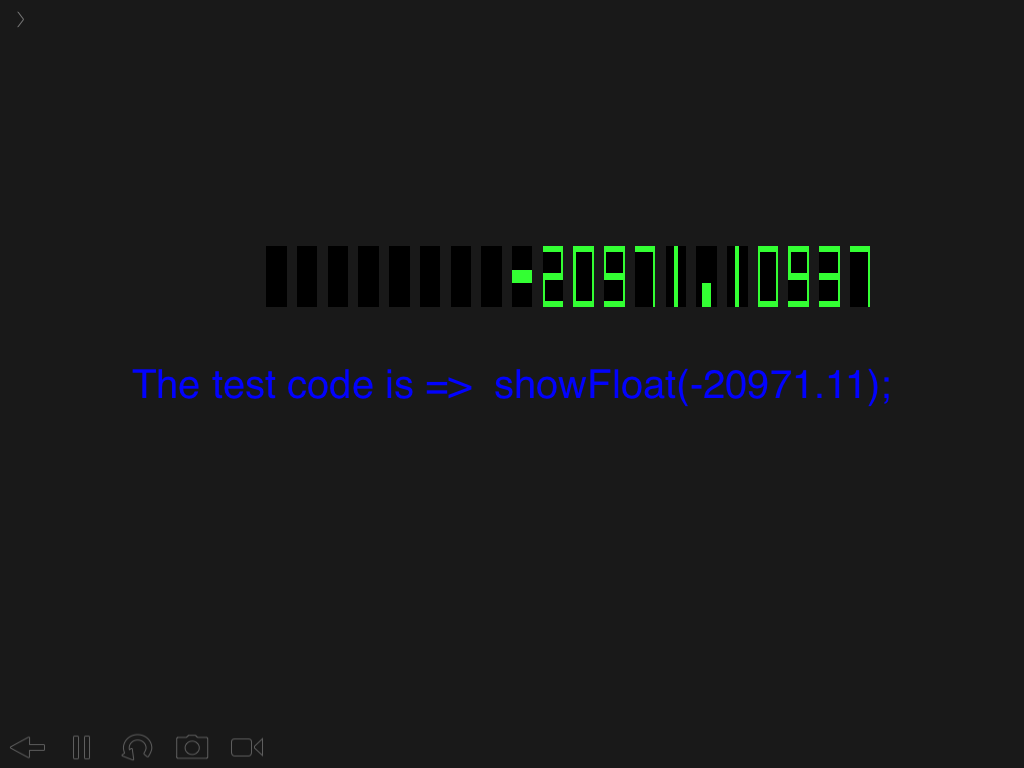#### Howdy, Stranger!

It looks like you're new here. If you want to get involved, click one of these buttons!

# How to watch a var in shader? -A new solution: change the question from What is to Is it

## Intro

### Description

When we program for `OpenGL ES 2.0 Shader` on `Codea`, we find that it is difficult to debug the var used in `shader`. The `GPU` is like a balck hole, programer can transfer data to the `vertex shader` and `fragment shader`, but has no method to fetch the value from `shader`, because of this we can not see the detail inside `shader`. When the `shader` has error, we have to guess and guess. The skill of debug -`print/optput`- can not take effact, so it is difficult to debug the `shader` code.

### What we have

At last the `shader` will output something, its output is two vars of `gl_Position` and `gl_FragColor`, the first is a vec4 used for setting the coordinate of pixel, the second is also a vec4 used for setting the color of pixel, but the two vars can not output the values of other vars what we want directlly. Then can we get a solution, to get the info undirectlly?

### Change our mind

Yesterday night when I was trying to write some simple but interesting `shader` code, suddenly I have an idea: Why not change the method of question to the `shader`?

### The 1st simple solution

It is easy to design the `shader` code which draw red color on specified area when the answer is `true`, and draw green color when the answer is `false`, then the `GPU` can give us the info what we need throughout the screen undirectlly.

Assume we want to watch the var `myVar`, want to know if it is bigger than 100, the `shader` code is below:

``````void main()

...

float x = vTexCoord.x;
float y = vTexCoord.y;

//  set the debug area is up/right corner
if(x > 0.9 && y > 0.9) {
if(myVar > 100){
// true is red
gl_FragColor = vec4(1,0,0,.5);
}else{
// false is green
gl_FragColor = vec4(0,1,0,.5);
}
}
}
``````

The whole `shader` code is below:

``````myShader = {
vsBase = [[
uniform mat4 modelViewProjection;
uniform vec2 uResolution;

attribute vec4 position;
attribute vec4 color;
attribute vec2 texCoord;

varying lowp vec4 vColor;
varying highp vec2 vTexCoord;

void main() {
vColor=color;
vTexCoord = texCoord;

gl_Position = modelViewProjection * position;
}
]],
fsBase = [[
precision highp float;
uniform lowp sampler2D texture;
varying lowp vec4 vColor;
varying highp vec2 vTexCoord;

void main() {
lowp vec4 col = texture2D( texture, vTexCoord ) * vColor;

// default is white
gl_FragColor = vec4(1,1,1,1);

// test var
int myVar = 1;

float x = vTexCoord.x;
float y = vTexCoord.y;

// debug area
if(x > 0.9 && x < 1.0 && y > 0.9 && y < 1.0) {
if(myVar > 100){
// true is red
gl_FragColor = vec4(1,0,0,1);
}else {
// false is green
gl_FragColor = vec4(0,1,0,1);
}
}
}
]]
}
``````

The `Codea` code is:

``````-- Shader debug
displayMode(OVERLAY)
function setup()
m = mesh()

-- m.texture = "Documents:univer"
m:setColors(color(220,200,200,255))

parameter.watch("m.vertices")

end

function draw()
background(0)
m:draw()
end

function touched(touch)

end

``````

• edited March 2016 Posts: 113

### The 2nd solution: Show the value

Very well, with the experiment above, we can know the brief of the var in `shader`. But the info is too simple, and it is complicated to use. We want to watch the exact value, we know we can control the whole screen with `shader`, so in abstracto we can draw anything to screen include number.

Now let us make the problem simple, assume `myVar` is a int and its region is [0,9], then we can get a logic:

``````if myVar is 1, then draw specified pixels in a specified area with specified color(draw 1);
if myVar is 2, then draw specified pixels in a specified area with specified color(draw 2);
...
if myVar is 9, then draw specified pixels in a specified area with specified color(draw 9);
if myVar is 0, then draw specified pixels in a specified area with specified color(draw 0);
``````

It looks good, now we can make `shader` output the 10 number of `1~0`. Continue to make the problem simpler, start with the easiest place, only to draw a number `1` on the screen, the code is below:

``````float x = vTexCoord.x;
float y = vTexCoord.y;

// debug area
if(x > 0.9 && x < 1.0 && y > 0.9 && y < 1.0) {
// background is white
gl_FragColor = vec4(1,1,1,1);
// draw 1 in a rect
if( x > 0.99 ){
// the right set green
gl_FragColor = vec4(0,1,0,1);
}
}
``````

Ok, it works, now we can add more, for example we can add a judgement with `if myVar is number 1`, if it is `true` the `shader` will draw a green number 1 with white background in this area.

``````float x = vTexCoord.x;
float y = vTexCoord.y;

// debug area
if(x > 0.9 && x < 1.0 && y > 0.9 && y < 1.0) {
// background is white
gl_FragColor = vec4(1,1,1,1);
// draw 1 in a rect
if( myVar == 1 && x > 0.99 ){
// set green
gl_FragColor = vec4(0,1,0,1);
}
}
``````

One by one, we can draw the number `2~0` with the same method:

``````float x = vTexCoord.x;
float y = vTexCoord.y;

// debug area
if(x > 0.9 && x < 1.0 && y > 0.9 && y < 1.0) {
//  background is white
gl_FragColor = vec4(1,1,1,1);
// draw 1 in a rect
if( myVar == 1 && x > 0.99 ){
// set green
gl_FragColor = vec4(0,1,0,1);
}
if( myVar == 2 && (the coordinate of 2) ){
// set green
gl_FragColor = vec4(0,1,0,1);
}
...
if( myVar == 0 && (the coordinate of 0) ){
// set green
gl_FragColor = vec4(0,1,0,1);
}
}
``````

Optimize the code:

``````float x = vTexCoord.x;
float y = vTexCoord.y;

// debug area
if(x > 0.9 && x < 1.0 && y > 0.9 && y < 1.0) {
// background is white
gl_FragColor = vec4(1,1,1,1);
// draw 1 in a rect
if(( myVar == 1 && x > 0.99 ) ||
( myVar == 2 && (the coordinate of 2)) ||
...
( myVar == 0 && (the coordinate of 0))
)
{
// set green
gl_FragColor = vec4(0,1,0,1);
}
}
``````

It is ok, change it to a function:

``````// LED char
void ledChar(int n, float xa,float xb, float ya, float yb){
float x = vTexCoord.x;
float y = vTexCoord.y;
float x1 = xa;
float x2 = xa+xb;
float y1 = ya;
float y2 = ya+yb;
float ox = (x2+x1)/2.0;
float oy = (y2+y1)/2.0;
float dx = (x2-x1)/10.0;
float dy = (y2-y1)/10.0;
float b = (x2-x1)/20.0;
int num = n;

// debug area
if(x >= x1 && x <= x2 && y >= y1 && y <= y2) {
// background is blue
gl_FragColor = vec4(0,0,1,.5);
// draw 1~0 in a rect
if((num==1 && (x > x2-dx)) ||
(num==2 && ((y > y2-dy) || (x > x2-dx && y > oy-dy/2.0) || (y > oy-dy/2.0 && y < oy+dy/2.0) || (x < x1+dx && y < oy+dy/2.0) || (y < y1+dy))) ||
(num==3 && ((y > y2-dy) || (x > x2-dx) || (y > oy-dy/2.0 && y < oy+dy/2.0) ||  (y < y1+dy))) ||
(num==4 && ((x < x1+dx && y > oy-dy/2.0) ||(x > x2-dx) || (y > oy-dy/2.0 && y < oy+dy/2.0))) ||
(num==5 && ((y > y2-dy) || (x < x1+dx && y > oy-dy/2.0)|| (y > oy-dy/2.0 && y < oy+dy/2.0) || (x>x2-dx && y <oy-dy/2.0) || (y<y1+dy))) ||
(num==6 && ((y > y2-dy) || (x < x1+dx)|| (y > oy-dy/2.0 && y < oy+dy/2.0) || (x>x2-dx && y <oy-dy/2.0) || (y<y1+dy))) ||
(num==7 && ((y > y2-dy) || (x > x2-dx))) ||
(num==8 && ((y > y2-dy) || (x < x1+dx)|| (y > oy-dy/2.0 && y < oy+dy/2.0) || (x>x2-dx) || (y<y1+dy))) ||
(num==9 && ((y > y2-dy) || (x < x1+dx && y > oy-dy/2.0)||(y > oy-dy/2.0 && y < oy+dy/2.0)|| (x>x2-dx) || (y<y1+dy))) ||
(num==0 && ((y > y2-dy) || (x < x1+dx) || (x>x2-dx) || (y<y1+dy)))
)
{
// set green
gl_FragColor = vec4(0,1,0,1);
}
}
}
``````

The whole `shader` code is below:

``````myShader = {
vsBase = [[
uniform mat4 modelViewProjection;
uniform vec2 uResolution;

attribute vec4 position;
attribute vec4 color;
attribute vec2 texCoord;

varying lowp vec4 vColor;
varying highp vec2 vTexCoord;

void main() {
vColor=color;
vTexCoord = texCoord;

gl_Position = modelViewProjection * position;
}
]],
fsBase = [[
precision highp float;
uniform lowp sampler2D texture;
varying lowp vec4 vColor;
varying highp vec2 vTexCoord;

void ledChar(int,float,float,float,float);

void main() {
lowp vec4 col = texture2D( texture, vTexCoord ) * vColor;

// default is white
gl_FragColor = vec4(.1,.1,.1,1);

// show 1
ledChar(1, 0.9, 0.1, 0.9, 0.1);
}

// LED char
void ledChar(int n, float xa,float xb, float ya, float yb){
float x = vTexCoord.x;
float y = vTexCoord.y;
float x1 = xa;
float x2 = xa+xb;
float y1 = ya;
float y2 = ya+yb;
float ox = (x2+x1)/2.0;
float oy = (y2+y1)/2.0;
float dx = (x2-x1)/10.0;
float dy = (y2-y1)/10.0;
float b = (x2-x1)/20.0;
int num = n;

// debug area
if(x >= x1 && x <= x2 && y >= y1 && y <= y2) {
// set blue
gl_FragColor = vec4(0.2,0.2,0.8,.5);
// draw number 1~0
if((num==1 && (x > x2-dx)) ||
(num==2 && ((y > y2-dy) || (x > x2-dx && y > oy-dy/2.0) || (y > oy-dy/2.0 && y < oy+dy/2.0) || (x < x1+dx && y < oy+dy/2.0) || (y < y1+dy))) ||
(num==3 && ((y > y2-dy) || (x > x2-dx) || (y > oy-dy/2.0 && y < oy+dy/2.0) ||  (y < y1+dy))) ||
(num==4 && ((x < x1+dx && y > oy-dy/2.0) ||(x > x2-dx) || (y > oy-dy/2.0 && y < oy+dy/2.0))) ||
(num==5 && ((y > y2-dy) || (x < x1+dx && y > oy-dy/2.0)|| (y > oy-dy/2.0 && y < oy+dy/2.0) || (x>x2-dx && y <oy-dy/2.0) || (y<y1+dy))) ||
(num==6 && ((y > y2-dy) || (x < x1+dx)|| (y > oy-dy/2.0 && y < oy+dy/2.0) || (x>x2-dx && y <oy-dy/2.0) || (y<y1+dy))) ||
(num==7 && ((y > y2-dy) || (x > x2-dx))) ||
(num==8 && ((y > y2-dy) || (x < x1+dx)|| (y > oy-dy/2.0 && y < oy+dy/2.0) || (x>x2-dx) || (y<y1+dy))) ||
(num==9 && ((y > y2-dy) || (x < x1+dx && y > oy-dy/2.0)||(y > oy-dy/2.0 && y < oy+dy/2.0)|| (x>x2-dx) || (y<y1+dy))) ||
(num==0 && ((y > y2-dy) || (x < x1+dx) || (x>x2-dx) || (y<y1+dy)))
)
{
// set green
gl_FragColor = vec4(0,1,0,1);
}
}
}
]]
}
``````

In abstracto, with the foundation code above, we can watch the var value in `shader` easily, but unfortunately there is a big `bug` I have not found the reason: when in one mesh run the function `ledChar` twice, it will make the screen messy.

About how to display number font with `shader`, I have thought of some other method, one is using `vec4` or `mat4` to transfer the font data , another is using the `texture`. I think the last one will be esaier. I will try these ideas later.

Btw, when I have had these ideas, I thought maybe others will have the same idea, so I searched for the keywords `shader debug`, then I found in `StackOverflow` some programers were talking about it, one person give the answer similar with the first solution of mine, and one person gave a solution full of imagination--visualization. It looks very interesting. The article linke, I will try the visualization later.

• Posts: 113

The screenshot of the 1st solution:
myVar > 100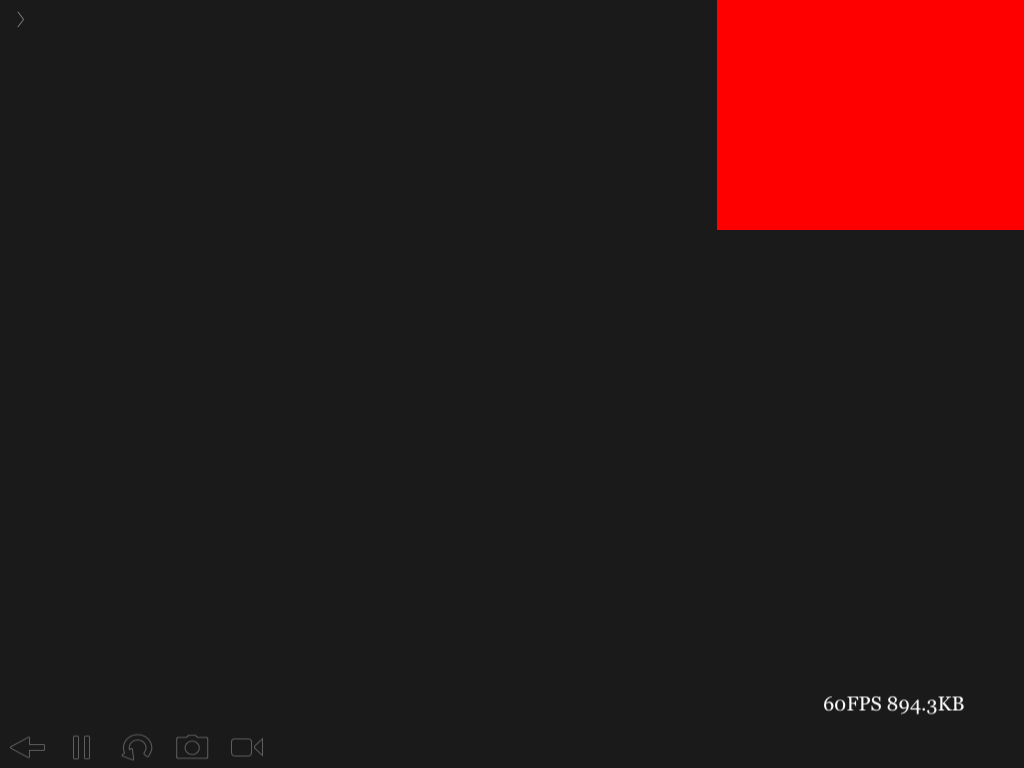myVar < 100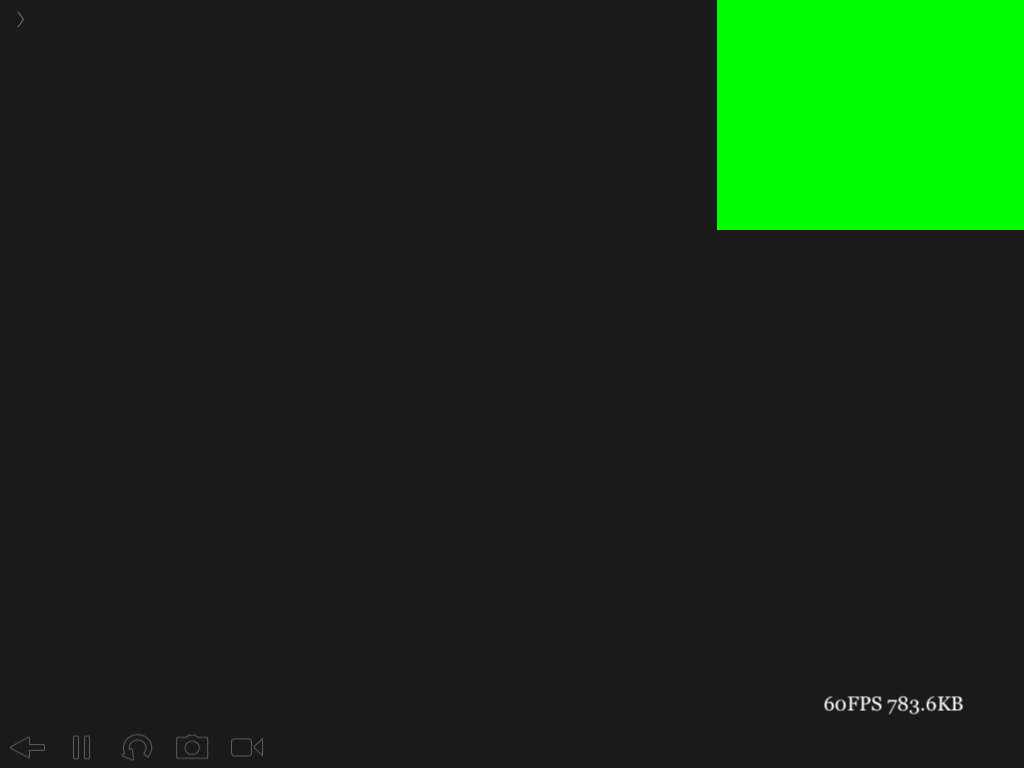The screenshot of the 2nd solution:
Number 4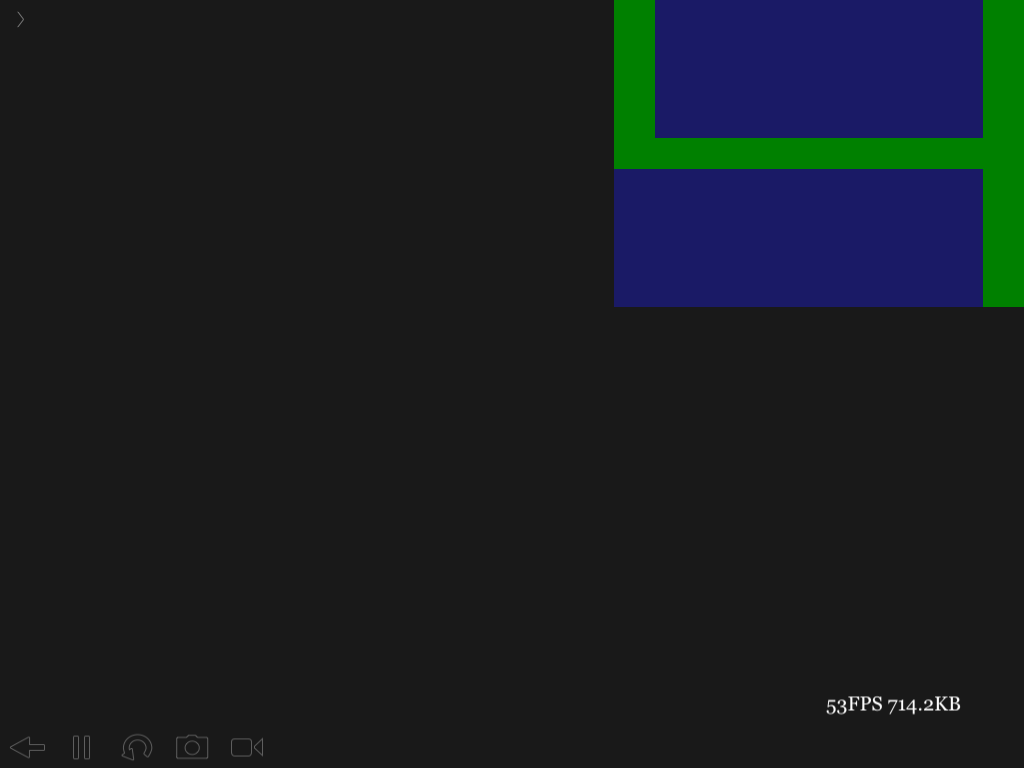Number 2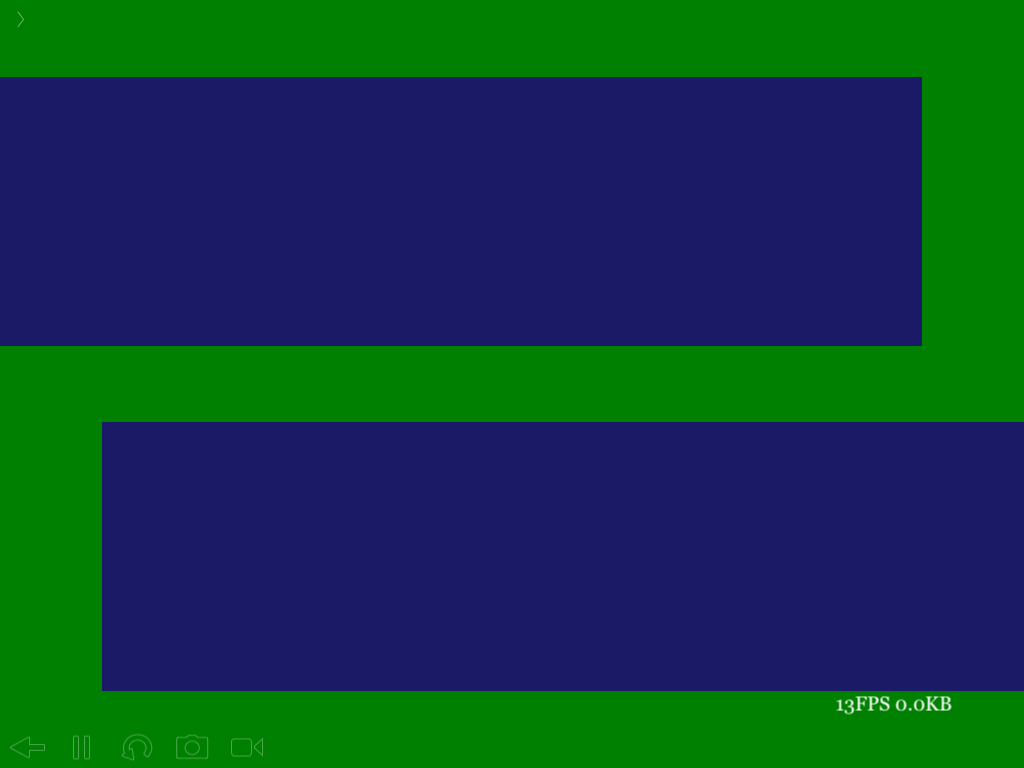• edited March 2016 Posts: 9,279

@binaryblues Nice trick to show a value. I changed your code a little, but I haven't played with shaders that much, so I couldn't do everything I wanted. Maybe you can finish this. The idea is to take a value and create a while loop to get each digit of the value and pass it to ledChar incrementing the xa value by .015 each time. I just called ledChar 6 times to show you what it would look like.

EDIT: I added the while loop to show the number and removed the 6 calls to ledChar.

``````displayMode(OVERLAY)

function setup()
m = mesh()
end

function draw()
background(0)
m:draw()
end

vsBase = [[ // vertex shader
uniform mat4 modelViewProjection;
uniform vec2 uResolution;
attribute vec4 position;
attribute vec4 color;
attribute vec2 texCoord;
varying lowp vec4 vColor;
varying highp vec2 vTexCoord;
void main() {
vColor=color;
vTexCoord = texCoord;
gl_Position = modelViewProjection * position;
}   ]],

fsBase = [[ // fragment shader
precision highp float;
uniform lowp sampler2D texture;
varying lowp vec4 vColor;
varying highp vec2 vTexCoord;
void ledChar(int,float,float,float,float);
void main()
{   lowp vec4 col = texture2D( texture, vTexCoord ) * vColor;
gl_FragColor = vec4(.1,.1,.1,1);

highp int nbr=856293;   // number to display

float m=0.96;
while (nbr>0)
{   m=m-0.015;
int nn=nbr-((nbr/10)*10);
ledChar(nn, m, 0.01, 0.96, 0.01);
nbr=nbr/10;
}
}
// LED char
void ledChar(int num, float xa,float xb, float ya, float yb)
{   float x = vTexCoord.x;
float y = vTexCoord.y;
float x1 = xa;
float x2 = xa+xb;
float y1 = ya;
float y2 = ya+yb;
float ox = (x2+x1)/2.0;
float oy = (y2+y1)/2.0;
float dx = (x2-x1)/6.0;
float dy = (y2-y1)/6.0;
// debug area
if(x >= x1 && x <= x2 && y >= y1 && y <= y2)
{   // set blue
gl_FragColor = vec4(0.2,0.2,0.8,.5);

// draw number 1~0
if  ((num==1 && (x > x2-dx)) ||
(num==2 && ((y > y2-dy) ||
(x > x2-dx && y > oy-dy/2.0) ||
(y > oy-dy/2.0 && y < oy+dy/2.0) ||
(x < x1+dx && y < oy+dy/2.0) || (y < y1+dy))) ||
(num==3 && ((y > y2-dy) || (x > x2-dx) ||
(y > oy-dy/2.0 && y < oy+dy/2.0) || (y < y1+dy))) ||
(num==4 && ((x < x1+dx && y > oy-dy/2.0) ||
(x > x2-dx) || (y > oy-dy/2.0 && y < oy+dy/2.0))) ||
(num==5 && ((y > y2-dy) ||
(x < x1+dx && y > oy-dy/2.0)||
(y > oy-dy/2.0 && y < oy+dy/2.0) ||
(x>x2-dx && y <oy-dy/2.0) || (y<y1+dy))) ||
(num==6 && ((y > y2-dy) || (x < x1+dx)||
(y > oy-dy/2.0 && y < oy+dy/2.0) ||
(x>x2-dx && y <oy-dy/2.0) || (y<y1+dy))) ||
(num==7 && ((y > y2-dy) || (x > x2-dx))) ||
(num==8 && ((y > y2-dy) || (x < x1+dx)||
(y > oy-dy/2.0 && y < oy+dy/2.0) ||
(x>x2-dx) || (y<y1+dy))) ||
(num==9 && ((y > y2-dy) || (x < x1+dx && y > oy-dy/2.0)||
(y > oy-dy/2.0 && y < oy+dy/2.0)|| (x>x2-dx) ||
(y<y1+dy))) ||
(num==0 && ((y > y2-dy) || (x < x1+dx) || (x>x2-dx) || (y<y1+dy))))
{   // set green
gl_FragColor = vec4(0,1,0,1);
}
}
}
]]
}
``````
• Posts: 5,396

Yes, using a colour to pass a message from a shader is something that is commonly used, because there is no other way to do it

• Posts: 113

@dave1707 thanks for your code and suggestion, I will make it a prototype on the base of your code .
@Ignatz It is hard to debug the shader code, so I have to left shader for a while...but now I am back.Now a new available but low FPS version is OK!

At first I updated the function, the judgement is hard to read, so I change it with a method which I call it "rect mask", see the below: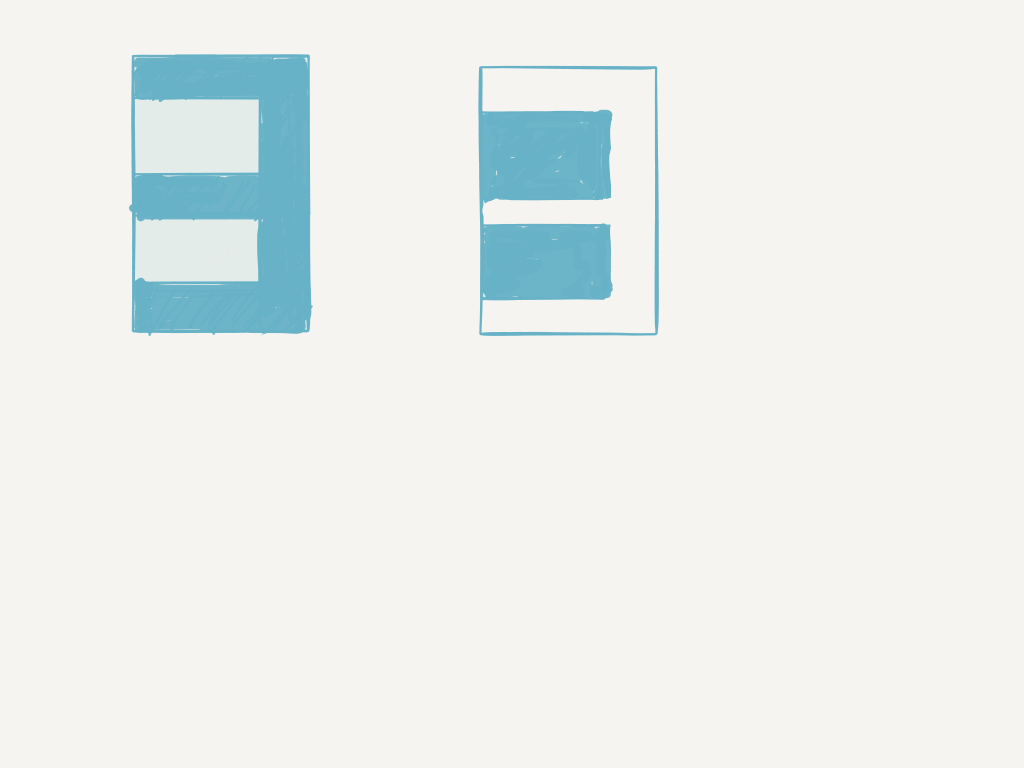The left one is the old code, I have to check 4 rect areas, the right one is the new, only need to check 2 rect areas, and it is easy to understand.
Then I add the code for dealing with float and negative. The whole code is below:

``````myShader = {
vsBase = [[
uniform mat4 modelViewProjection;
uniform vec2 uResolution;

attribute vec4 position;
attribute vec4 color;
attribute vec2 texCoord;

varying lowp vec4 vColor;
varying highp vec2 vTexCoord;

void main() {
vColor=color;
vTexCoord = texCoord;

gl_Position = modelViewProjection * position;
}
]],
fsBase = [[
precision highp float;
uniform lowp sampler2D texture;
varying lowp vec4 vColor;
varying highp vec2 vTexCoord;

float x = vTexCoord.x;
float y = vTexCoord.y;

void ledChar(int,float,float,float,float);
void ledRectChar(int,float,float,float,float);
void showInt(int);
void showFloat(float);
bool inRect(float,float,float,float);

void main() {
lowp vec4 col = texture2D( texture, vTexCoord ) * vColor;

// 默认全部设置为黑色
gl_FragColor = vec4(.1,.1,.1,1);

showFloat(-.1111111);
//showFloat(float(-9765));

}

void showFloat(float f){
int myNum;
int k = 0;
int iPart = int(floor(abs(f)));
int fPart = int(fract(abs(f))*100000.0);
float m=0.86;

// 初始化数组,全部置为代表黑色的12
for(int i=0; i<20; i++){
myNum[i] = 12;
}

// 插入小数部分
while (fPart>0)
{
// 从个位开始, 依次取出个位,十位,百位,千位...的数字值
myNum[k++]=fPart-((fPart/10)*10);
fPart=fPart/10;
}

// 如果是0
if(f==0.0){myNum[k++] = 0;}

// 插入小数点
myNum[k++] = 10;

// 插入整数部分
while (iPart>0)
{
myNum[k++]=iPart-((iPart/10)*10);
iPart=iPart/10;
}

// 如果是负数,则插入代表负号的11
if(f<0.0) { myNum[k++]=11;}

// 循环输出数字数组
for(int i=0; i<20; i++)
{
m = m-0.03;
ledRectChar(myNum[i], m, 0.02, 0.6, 0.15);
}
}

bool inRect(float x1,float x2, float y1, float y2){
if(x>x1 && x<x2 && y>y1 && y<y2) { return true; } else { return false; }
}

void ledRectChar(int n, float xa,float xb, float ya, float yb){
float x1 = xa;
float x2 = xa+xb;
float y1 = ya;
float y2 = ya+yb;
float ox = (x2+x1)/2.0;
float oy = (y2+y1)/2.0;
float dx = (x2-x1)/10.0;
float dy = (y2-y1)/10.0;
float b = (x2-x1)/20.0;
int num = n;

// 设定调试区显示范围
if(x >= x1 && x <= x2 && y >= y1 && y <= y2) {
// 设置调试区背景色
gl_FragColor = vec4(0.2,1.0,0.2,1.0);
// 分别绘制出 LED 形式的数字 1~0 , 用黑色绘制1个或2个矩形,由矩形以外的绿色区域组成字型
if((num==1 && (inRect(x1,ox-dx,y1,y2) || inRect(ox+dx,x2,y1,y2))) ||
(num==2 && (inRect(x1,x2-dx,oy+dy/2.0,y2-dy) || inRect(x1+dx,x2,y1+dy,oy-dy/2.0))) ||
(num==3 && (inRect(x1,x2-dx,oy+dy/2.0,y2-dy) || inRect(x1,x2-dx,y1+dy,oy-dy/2.0))) ||
(num==4 && (inRect(x1+dx,x2-dx,oy+dy/2.0,y2) || inRect(x1,x2-dx,y1,oy-dy/2.0))) ||
(num==5 && (inRect(x1+dx,x2,oy+dy/2.0,y2-dy) || inRect(x1,x2-dx,y1+dy,oy-dy/2.0))) ||
(num==6 && (inRect(x1+dx,x2,oy+dy/2.0,y2-dy) || inRect(x1+dx,x2-dx,y1+dy,oy-dy))) ||
(num==7 && inRect(x1,x2-dx,y1,y2-dy)) ||
(num==8 && (inRect(x1+dx,x2-dx,oy+dy/2.0,y2-dy) || inRect(x1+dx,x2-dx,y1+dy,oy-dy/2.0))) ||
(num==9 && (inRect(x1+dx,x2-dx,oy+dy/2.0,y2-dy) || inRect(x1,x2-dx,y1+dy,oy-dy/2.0))) ||
(num==0 && inRect(x1+dx,x2-dx,y1+dy,y2-dy)) ||
// 传入10则绘制小数点, 传入11则绘制负号, 传入12则清空
(num==10 && (inRect(x1,x2,oy-dy,y2) || inRect(x1,ox-dx*2.0,y1,oy-dy) || inRect(ox+dx*2.0,x2,y1,oy-dy) )) ||
(num==11 && (inRect(x1,x2,oy+dy,y2) || inRect(x1,x2,y1,oy-dy))) ||
(num==12)
)
{
gl_FragColor = vec4(0,0,0,.5);
}
}
}

]]
}
``````

The screenshot